Your data analysis solution

# Using differencing to obtain a stationary time series

2017-10-20

This tutorial will help you describing a time series and transforming it so that it becomes stationary, in Excel using the XLSTAT software.

## Dataset for the differencing transformation

An Excel sheet with both the data and results can be downloaded by clicking here.

The data have been obtained in [Box, G.E.P. and Jenkins, G.M. (1976). Time Series Analysis: Forecasting and Control. Holden-Day, San Francisco], and correspond to monthly international airline passengers (in thousands) from January 1949 to December 1960. It is widely used as a non-stationary seasonal time series.

Our goal is to show how helpful a descriptive analysis can be prior to a modeling approach.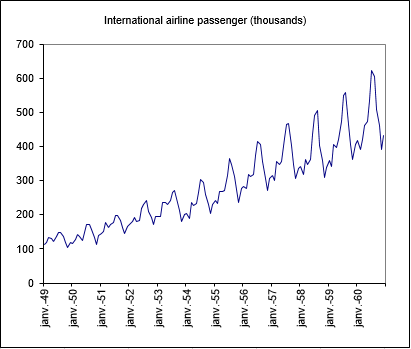We notice a global upward trend on the chart. Every year, a similar cycle starts while the variability within a year seems to increase over time. In order to confirm this trend we are going to analyze the autocorrelation function of the series.

## Setting up a descriptive analysis of time series

After opening XLSTAT, select the XLSTAT / Time / Descriptive analysis command.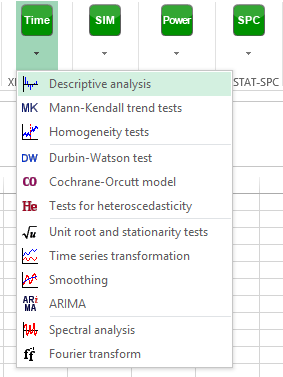Once you've clicked on the button, the Descriptive analysis dialog box appears. Select the data on the Excel sheet. The Time series corresponds to the series of interest, the Passengers. The option Series labels is activated because the first row of the selected data contains the header of the variable.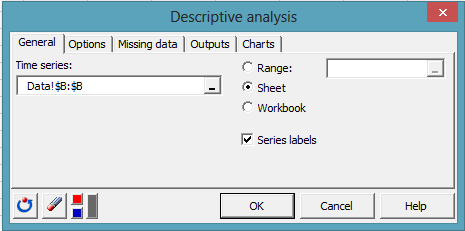In the Options tab, automatic time steps are selected: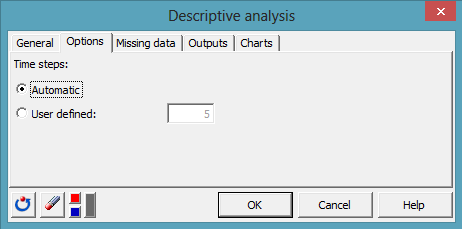The Outputs and Charts tabs are parameterized as follows: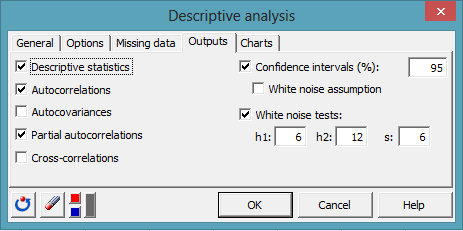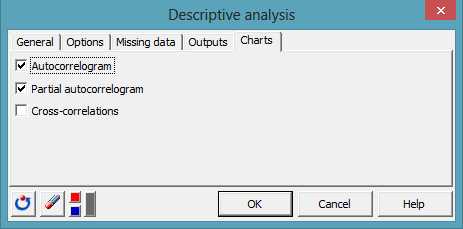The computations begin once you have clicked on OK. The results are then displayed.

## Interpreting the descriptive statistics of a time series

The first table displays the summary statistics. Then the Normality test and white noise tests table is displayed. The Jarque-Bera test is a normality test, based on the skewness and kurtosis coefficients. The higher the value of the Chi-square statistic, the more unlikely the null hypothesis that the data are normally distributed. Here the p-value, which corresponds to the probability of being wrong when rejecting the null hypothesis, is close to 0.012. With an alpha=0.05 significance level, one should reject the null hypothesis.

The three other three tests (Box-PierceLjung-BoxMcLeod-Li) are computed at different time lags. They allow to test if the data could be assumed to be a white noise or not. These tests are also based on the Chi-square distribution. They all agree that the data cannot be assumed to be generated by a white noise process. While the sorting of the data has no influence on the Jarque-Bera test, it does have an influence on the three other tests which are particularly suited for time series analysis.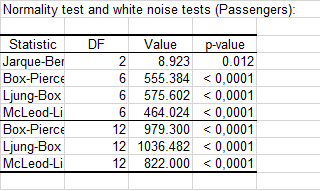Below the table that displays the descriptive functions of the time series, two bar charts display the evolution of the autocorrelation function (ACF)and of the partial autocorrelation function (PACF). The 95% confidence intervals are also displayed. By looking at the autocorrelogram, we can identify a clear lag 1 autocorrelation, as well as a seasonality which seems to be of 12 months.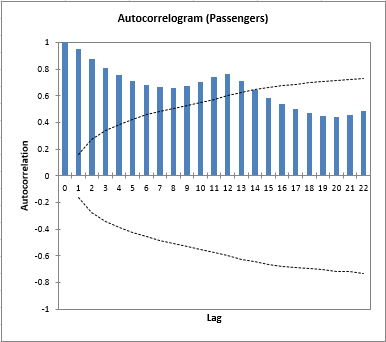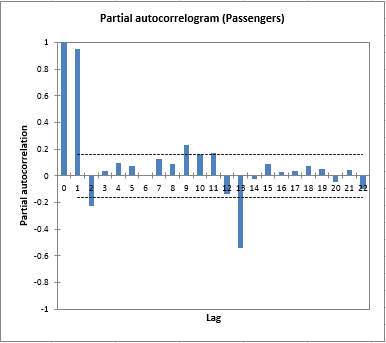## Transformation of a time series

In order to improve the normality of the data, we want to perform two transformations:

First, we want to stabilize the increasing variability of the series. Second, we want to remove the autocorrelations by differencing the series.

## Setting up the transformation of a time series

This can be done using the Time series transformation tool. To activate the corresponding dialog box, select the XLSTAT / XLSTAT-Time / Transforming series command, or click on the corresponding button of the XLSTAT-Time toolbar (see below).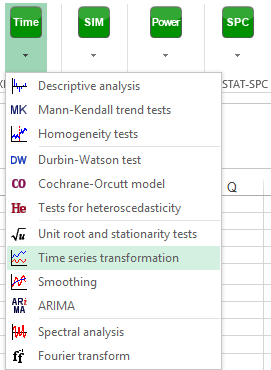Once you've clicked on the button, the dialog box appears.

Select the data on the Excel sheet. The Time series corresponds to the series of interest, the Passengers.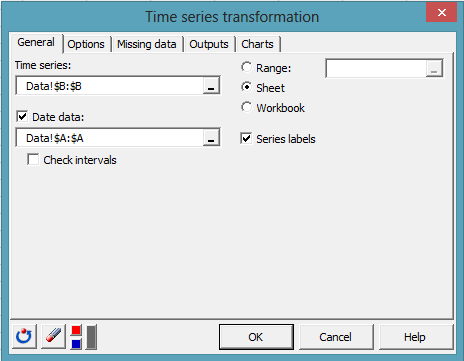After you selected the data, select the Box-Cox option in the Options tab.

We have the possibility to ask for an optimized transformation (the lambda parameter of the Box-Cox transformation would be adjusted so that the likelihood of a regression model - transformed Y = simple linear function of time - would be as high as possible). However, we decide here to fix the lambda value to 0, which corresponds to a log transformation of the series.

The log transformation is often a good choice for removing increasing variability.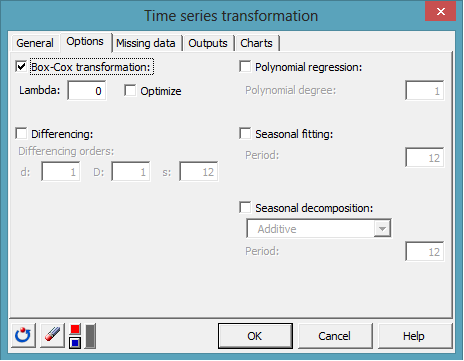The computations begin once you have clicked on OK.

## Results of the transformation of a time series

We first see a table and two charts: one for the original data set and the other for the Box-Cox transformation. As expected, the log transformation has removed the increasing variability.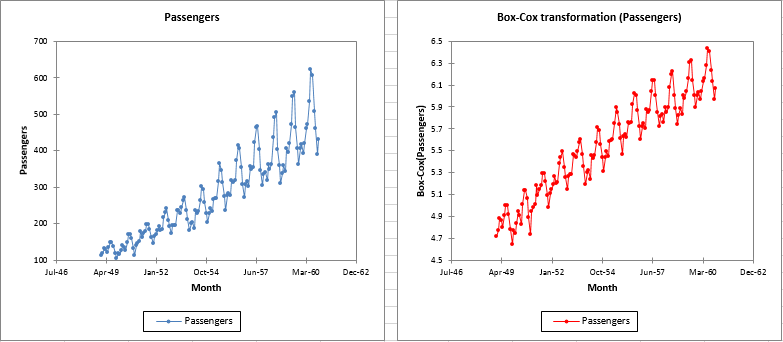Then, in order to remove the trend and the seasonal component, we decide to use the differencing method. We first select the Box-Cox transformed series on the new sheet.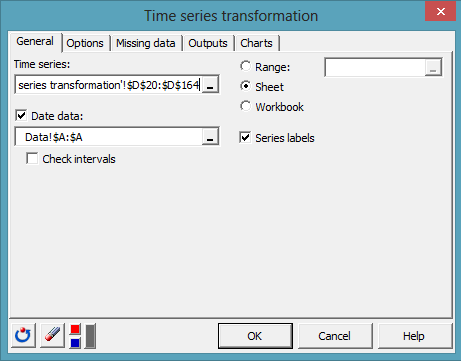We set the d value to 1 to remove the trend, and D and s to 1 and 12 to remove the 12 months seasonal component.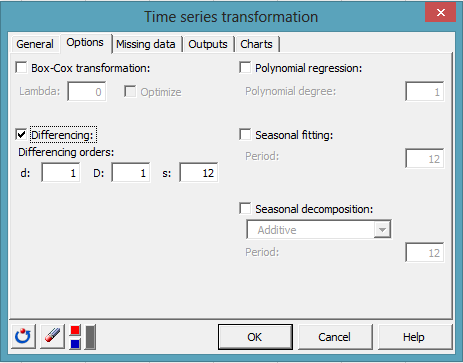The resulting chart shows that the differencing transformation effectively removed the trend.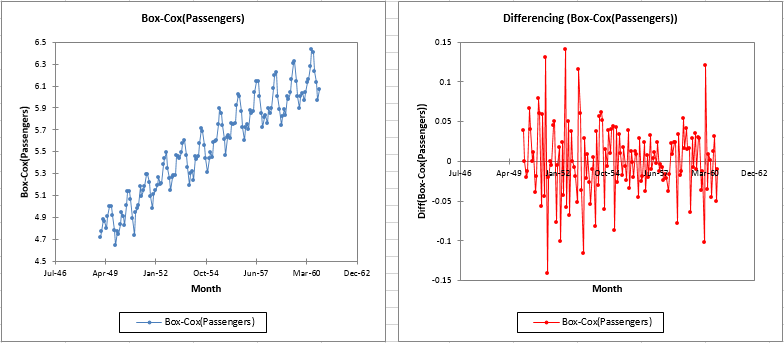## Descriptive statistics on transformed time series

We may check now if the differentiated series is a white noise by applying our descriptive analysis once again as shown on the figure.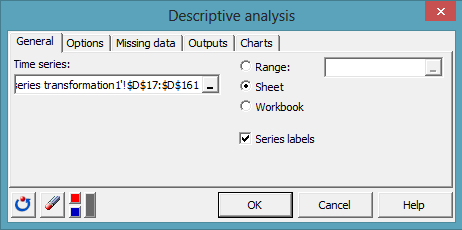As the differencing method created some missing values, we should decide how to handle them. In the Missing data tab, we activate the  Remove the observations option.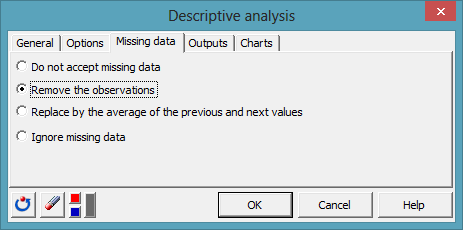The Jarque-Bera test confirms that the series gets closer to a normal sample (we went from 0.012 to 0.027) but remains not stationary as confirmed by the white noise tests.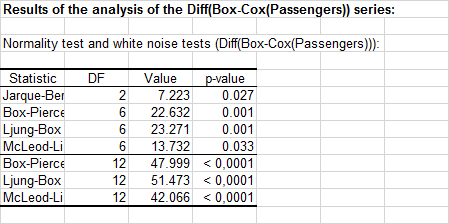Transformations have not been efficient enough. Indeed, the autocorrelogram indicates that some significant component remains at lag 1 and 12. Further investigations are needed in order to understand the underlying phenomenon.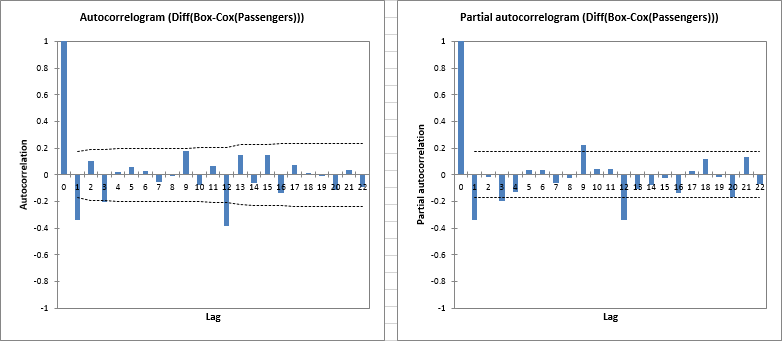## Seasonal decomposition of the series

Another approach to explore our time series would be to first decompose it into identified component using the Seasonal Decomposition option of the transformation tool. So we start again from the original data set as shown on the following figure.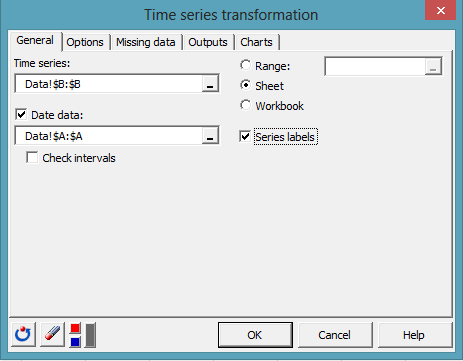This time, the Seasonal decomposition is selected in the Options tab. A multiplicative model seems appropriate as the time series exhibits a clear multiplicative behavior on the natural scale. The period is set to 12 for a 1-year periodicity on monthly data.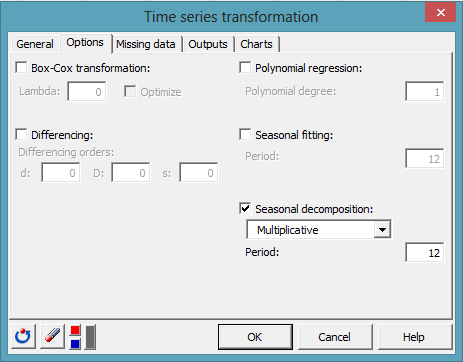Once computed, the decomposition is displayed via 4 plots: the original series, a trend component, a seasonal component and a random component. The last 3 series can be multiplied to each other in order to reconstruct the original series.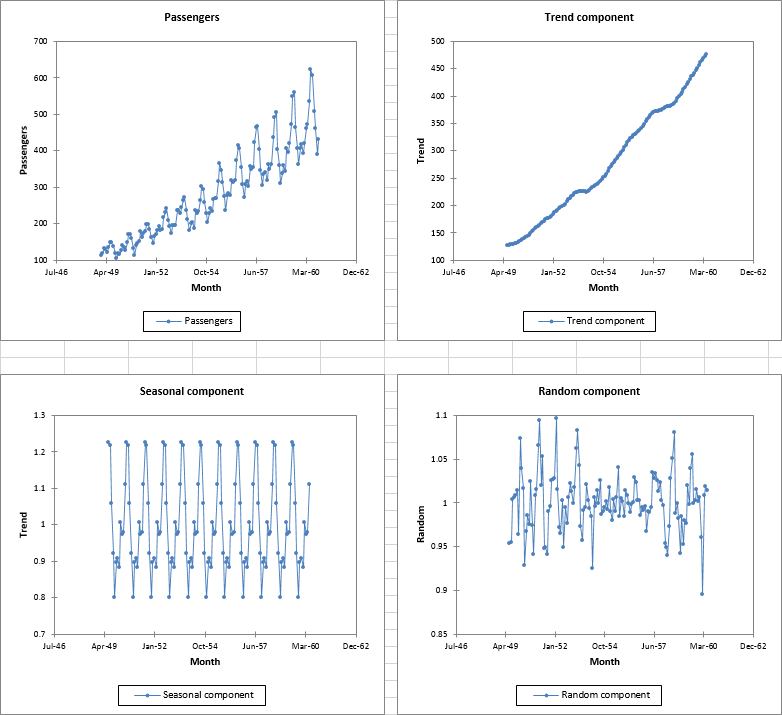The stationarity of the Random component might be tested now. However, we may first transform again this Random component using the Box-Cox transformation (log transformation) so that it is centered on 0.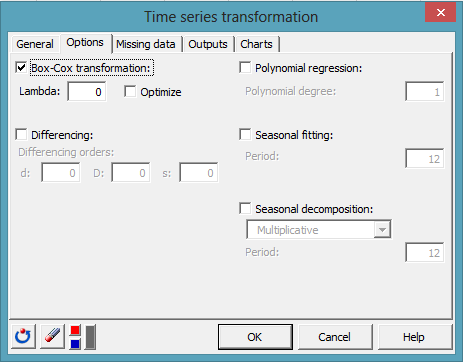Considering the resulting series displayed on the sheet.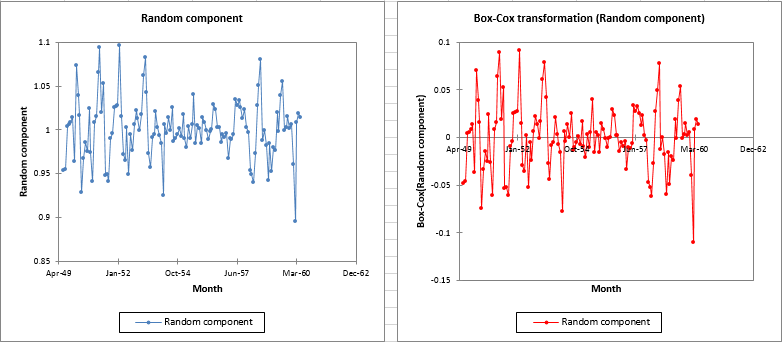The descriptive analysis is launched again on this series.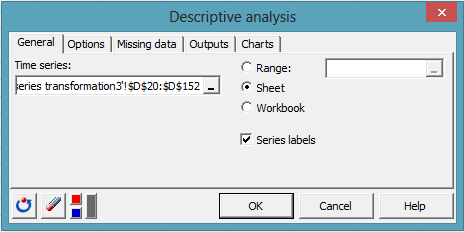This time the Jarque-Bera test does not allow to reject the hypothesis of a normally distributed variable.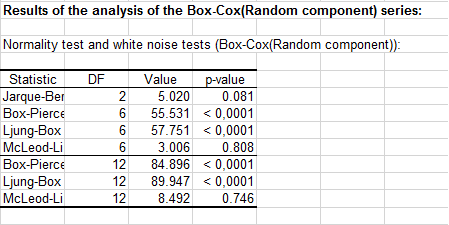Unfortunately, a seasonal pattern, less significant than before, remains visible in the autocorrelogram plot. This would call again for some further work on the generating process.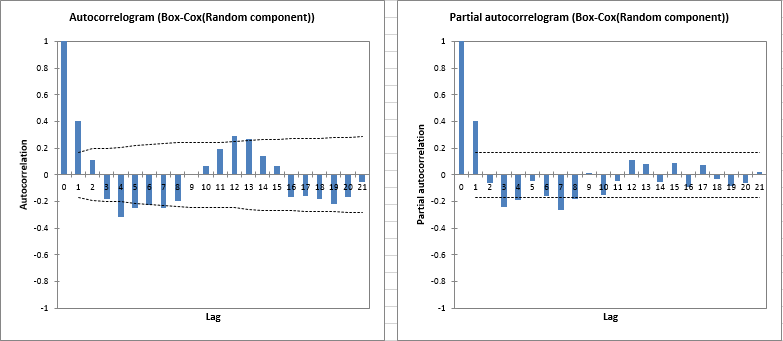1c26995d494fb3061dd0ae8571ffc0a4@xlstat.desk-mail.com
https://cdn.desk.com/
false
desk